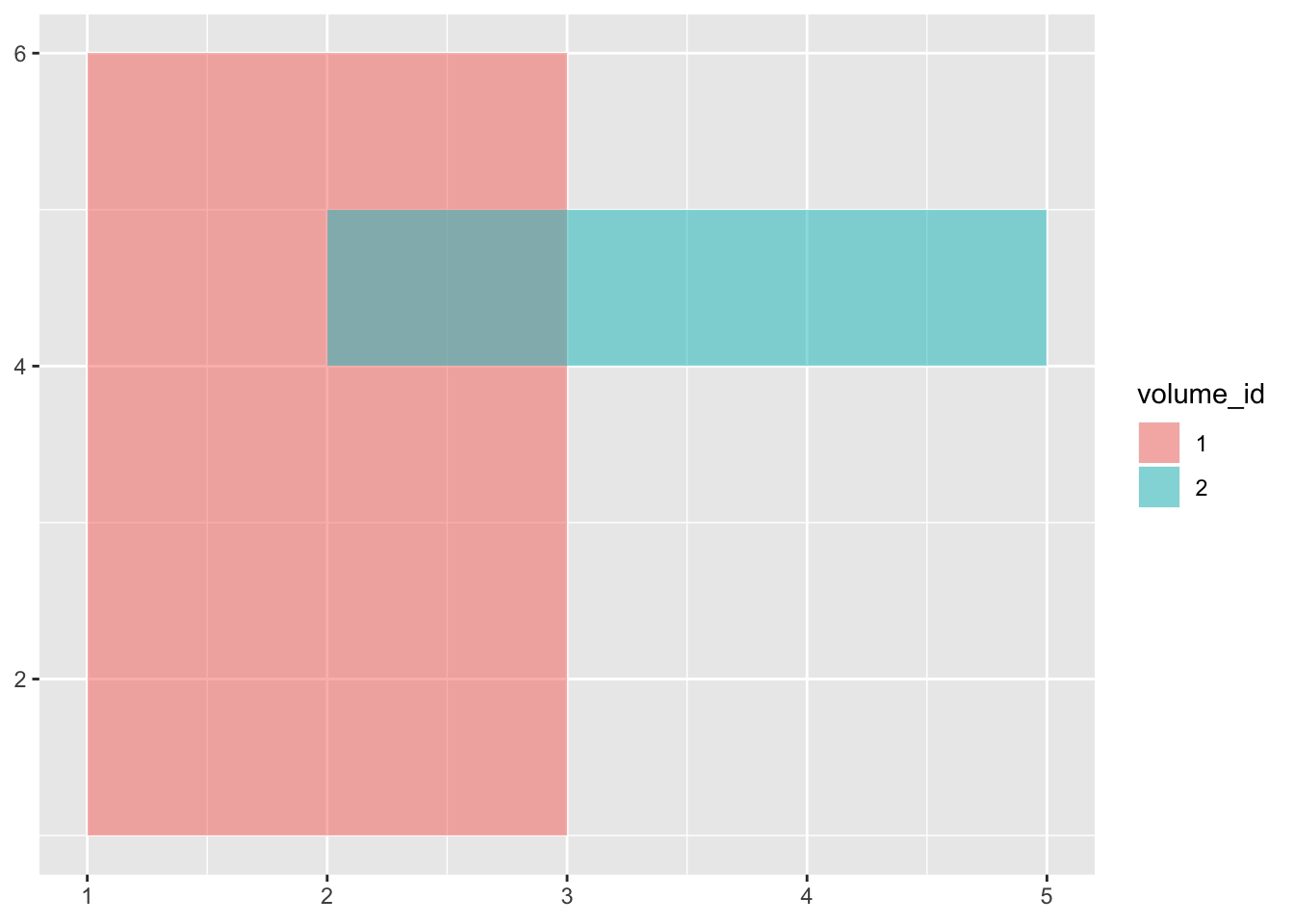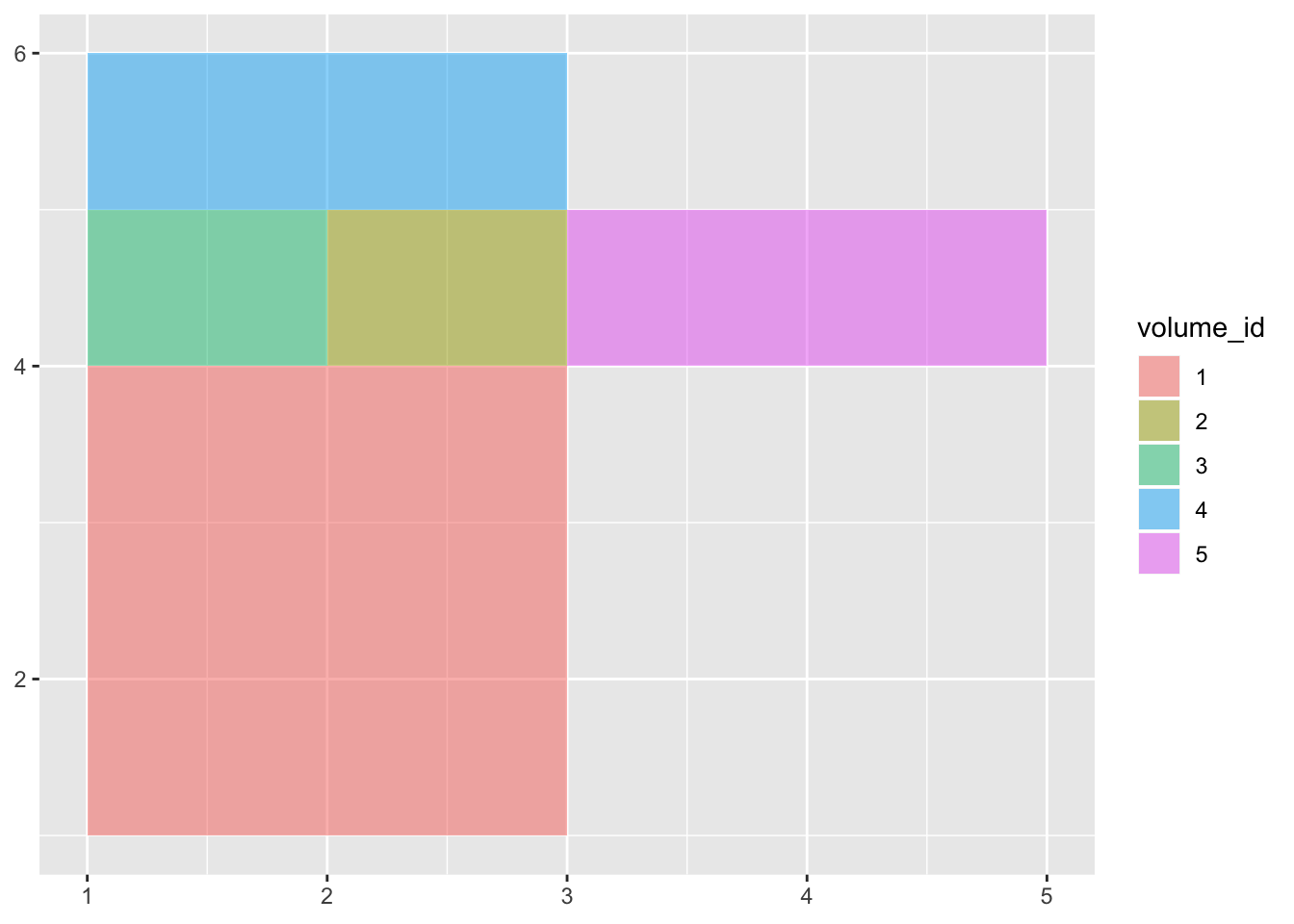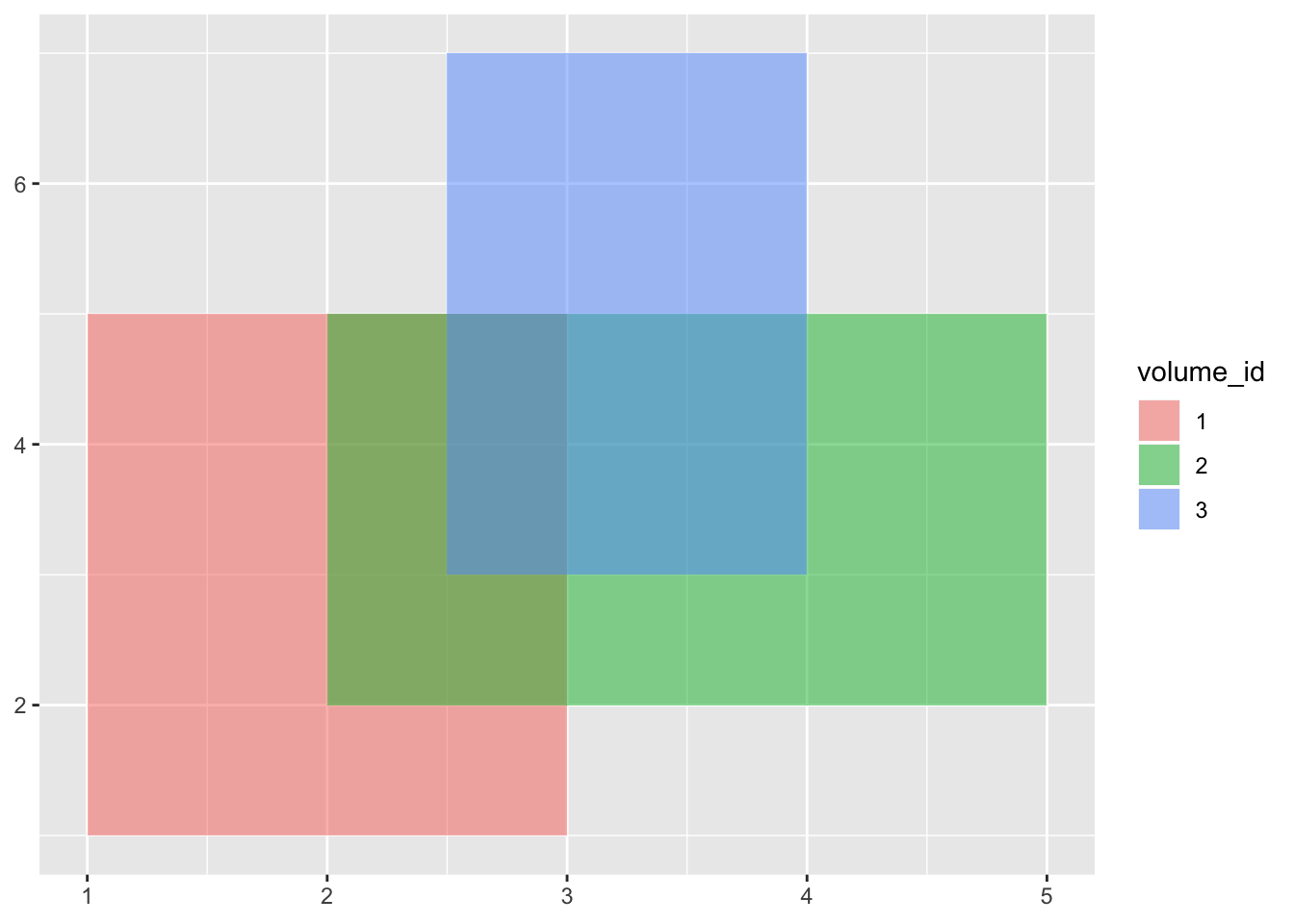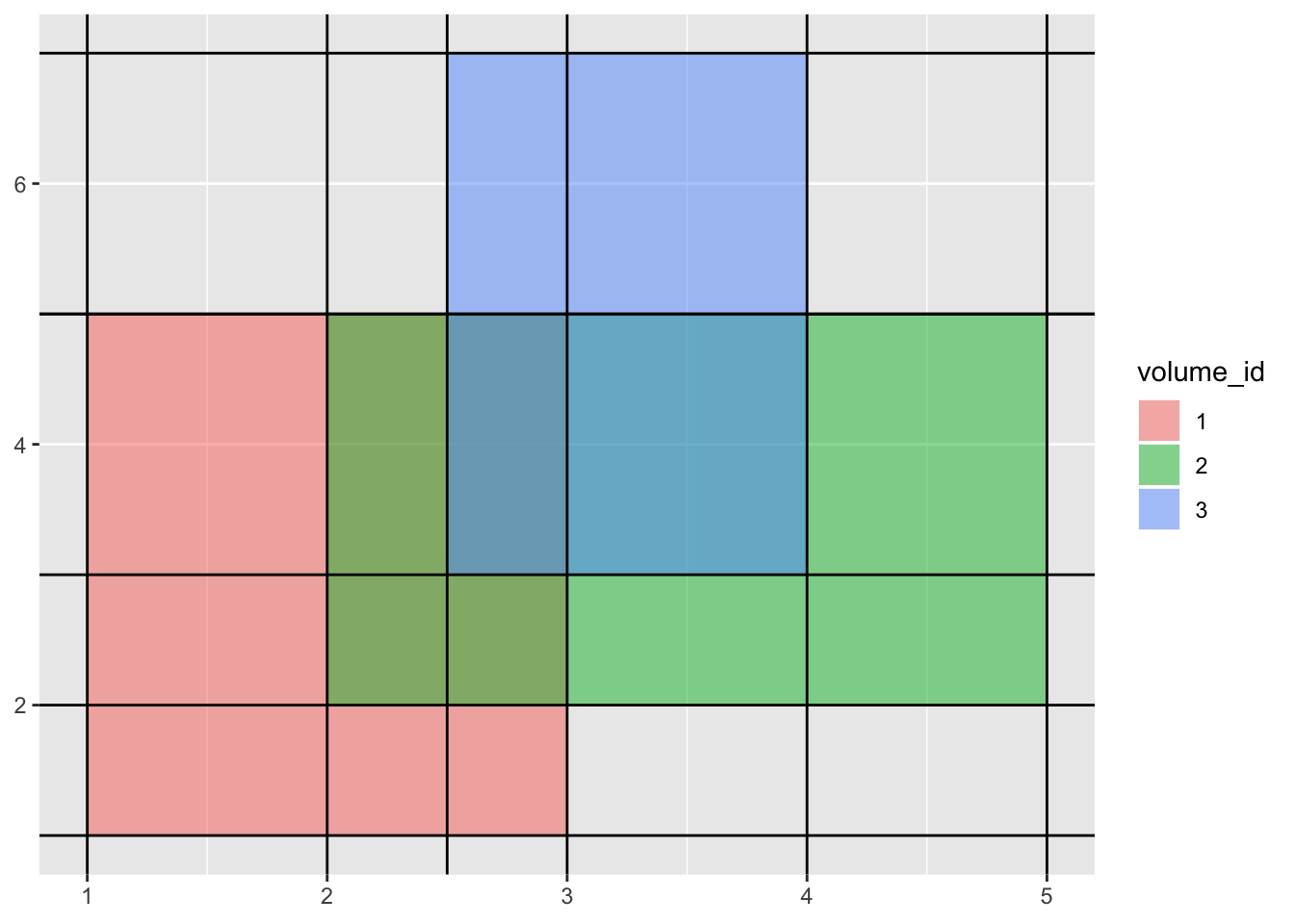## The Problem

First, a concise definition: Given a set of hyperrectangles, find any set of hyperrectangles which occupy the same space with no overlap & contain at least all the original boundaries. A hyperrectangle is the space defined by the cartesian product of ranges. With less jargon, axis-aligned rectangles/prisms/volumes in arbitrary dimensions.

Take an example - 2 dimension rectangles with some overlap:Here’s a solution:This is a valid solution because:

• the orginal space is exactly covered
• all the covering volumes are rectangles
• there is no overlap between rectangles
• none of the original boundaries are crossed in the solution.

### Motivation

Before describing a solution to this (possibly vacuous seeming) problem, I’d like to motivate it with an example.

The RuleFit algorithm (my implementation here) is a predictive modeling method. At a very high level, its purpose is to produce a set of conjunctive ranges on the predictor set (imagine them as a set of real valued vectors) which explain variation in the response vector. Take for example a predictor set of {age, weight, height} with a response of jumping height (i.e. we’re predicting how high someone can jump). One might imagine that lower age, lower weight, and larger height produce larger jumping height, and vice versa; RuleFit may pick up on such a pattern & produce, for example, the following set of “rules”:

• {height:[130-200] & weight:[50-60] & age:[10-25]}
• {height:[175-225] & weight:[55-75] & age:[20-35]}
• {height:[100-250] & weight:[70-100] & age:[45-55]}
• etc.

with the idea that a person qualifying for one or more of these rules provides useful information for predicting jumping height. In reality, each rule is assigned a real value (“effect”) which are summed up to produce the jumping height prediction.

Notice that many of these ranges are overlapped, which means a person may qualify for many rules. That makes interpretation & inference somewhat challenging, since we cannot longer can glance at a single rule as a single “nugget” of information. The practitioner is forced to contextualize rules & compute the high-dimensional intersections, which a human mind (or at least mine) isn’t good at. Wouldn’t it be great if these rules were disjoint, so that a person can only qualify for one rule? Solving the overlapped hyperrectangle problem does just that.

(Note: I’ve made some over-simplifications of RuleFit and encourage you to check out the above link if you’re interested.)

## Solution

A conceptually simple solution is to:

• Extend all boundaries to infinity (treating the boundary hyperplanes as fixed and allowing all other dimensions to be free)
• Re-create hyperrectangles according to the new boundaries
• Prune hyperrectangles outside the original covered space

To prove it’s a solution:

• It creates hyperrectangles, as all original boundaries are orthogonal by problem definition.
• Clearly all the original boundaries are preserved because they are simply scaled in size.
• It is capable of capturying a superset of the original space because it is partitioning the entire n-dimensional space.
• The non-covered hyperrectangles are able to be cleanly removed, as in without eating into the covered space, since, as argued above, all the original boundaries are preserved.

Without ado, we step into some code.

### Representation

Hyperrectangles will be represented in dimension-range format. In this representation, an n-dimensional hyperrectangle consists of n dimension-ranges. For this exercise, hyperrectangles are given an id and all placed into one data frame. Here we define and visualize a 2 dimensional example:

``````example_2d <- data.frame(
dimension = rep(c('x','y'), 3),
volume_id = rep(1:3, each=2),
min = c(1,1,
2,2,
2.5,3),
max = c(3,5,
5,5,
4,7)
) ``````### Extending Boundaries

This is as simple as picking “dimension=value” as a fixed point (while letting all other dimensions be free) for all range bounds.

``````build_fully_partitioned_space <- function(volumes) {
volumes %>%
mutate(bound = min) %>%
select(dimension, bound) %>%
rbind(
volumes %>%
mutate(bound = max) %>%
select(dimension, bound),
stringsAsFactors = FALSE
)
}``````

Visualizing our example:### Recreating Hyperrectangles

The next step is to take the orthogonal hyperplanes from the above step and form then into hyperrectangles abutted against one another.

``````generate_volumes_from_partitioned_space <- function(partitioned_space, id_starter = 1) {
if (nrow(partitioned_space) == 0) {
return(data.frame())
}

# pick an arbtirary first dimension
dimension_of_interest <- partitioned_space\$dimension
dimension_bounds <- partitioned_space %>%
filter(dimension == dimension_of_interest) %>%
# this is a small optimization - equal bounds are redundant
distinct() %>%
arrange(bound)

# there should always be 2 or more, since each bound corresponds to hyperrectangle edge
stopifnot(nrow(dimension_bounds) > 1)

# subspace meaning everything outside the dimension of interest
partitioned_subspace <- partitioned_space %>% filter(dimension != dimension_of_interest)
# recursively build ranges from the subspace before tacking on ranges for the dimension of interest in this stack frame
subspace_volumes <- generate_volumes_from_partitioned_space(partitioned_subspace, id_starter = id_starter)

# "expanded" by the dimension of interest, that is
expanded_volumes <- data.frame()
for (bound_ix in 1:(nrow(dimension_bounds) - 1)) {
# note that we are iterating on the sorted bounds
lower_bound <- dimension_bounds\$bound[bound_ix]
upper_bound <- dimension_bounds\$bound[bound_ix + 1]

if (nrow(subspace_volumes) == 0) {
# case this is the first dimension - there's nothing to add onto
volume_id <- paste0(id_starter, '_', dimension_of_interest, '_', bound_ix)
new_dimension_bounds <- list(volume_id = volume_id,
min = lower_bound,
max = upper_bound,
dimension = dimension_of_interest)
}
else {
# case this is after the first dimension - create a new volume for each subspace volume with the new bounds added (cartesian product)
new_dimension_bounds <- lapply(unique(subspace_volumes\$volume_id), function(volume_id) {
list(volume_id = paste0(volume_id, '_', dimension_of_interest, '_', bound_ix), # TODO this form of creating an ID could get costly in higher dimensions
min = lower_bound,
max = upper_bound,
dimension = dimension_of_interest)
}) %>% bind_rows() %>%
rbind(subspace_volumes %>%
mutate(volume_id = paste0(volume_id, '_', dimension_of_interest, '_', bound_ix)),
stringsAsFactors= FALSE)
}

expanded_volumes <- rbind(expanded_volumes, new_dimension_bounds,
stringsAsFactors = FALSE)
}

return(expanded_volumes)
}``````

Visualizing our results (with some difficulty, there’s a lot of colored rectangles):